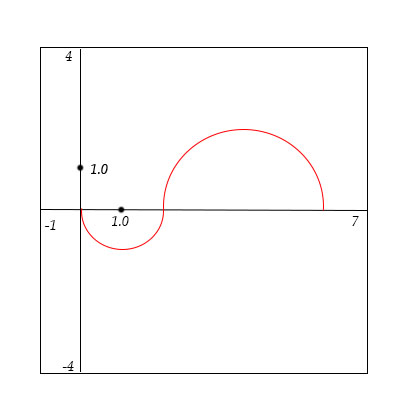# Evaluate the integrals for f(x) shown in the figure below. The two parts of the graph are...

## Question:

Evaluate the integrals for {eq}f(x) {/eq} shown in the figure below. The two parts of the graph are semicircles.

a. {eq}\int_0^2 4 f(x) dx {/eq}

b. {eq}\int_0^6 5 f(x) dx {/eq}

c. {eq}\int _1^4 3 f(x) dx {/eq}

d. {eq}\int _1^6|5f(x)|dx {/eq}## Finding Values of Definite Integrals Using Areas

Given the graph of a function which is comprised of two semi-circles, one above and the other below the x-axis, we use the concept from Calculus of the definite integral of a function being the positive or negative area of the region between the graph of the function and the x-axis to calculate several given definite integrals. The other concept being used here from Geometry is that the area of a circle is {eq}\pi r^2 {/eq} where {eq}r {/eq} is its radius. Hence the area of a semicircle and a quadrant of a circle would be one-half and one-quarter of the area of a circle, respectively.

To evaluate the definite integrals below, we use the following concepts from Calculus and Geometry:

(i) Definite integral of a function is the positive or negative area of the region between the graph of the function.

(ii) Linear Properties of Definite Integrals.

(iii) The area of a circle is {eq}\pi r^2 {/eq} where {eq}r {/eq} is its radius. Hence the area of a semicircle and a quadrant of a circle would be one-half and one-quarter of the area of a circle, respectively.

(a) {eq}\displaystyle \int_0^2 4 f(x) \; dx = 4 \int_0^2 f(x) \; dx = 4 \left( - \frac {\pi \; 1^2}{2} \right) = -2\pi. {/eq}

(b) {eq}\displaystyle \int_0^6 5 f(x) \; dx = 5 \left( \int_0^2 f(x) \; dx + \int_2^6 f(x) \; dx \right) = 5 \left( -\pi/2 + \pi (2^2)/2 \right) = 5 (2 \pi - \pi/2) = \frac {15\pi}{2}. {/eq}

(c) {eq}\displaystyle \int_1^4 3 f(x) \; dx = 3 \left( \int_1^2 f(x) \; dx + \int_2^4 f(x) \; dx \right) = 3 \left( -\pi/4 + \pi (2^2)/4 \right) = 3 (\pi - \pi/4) = \frac {9\pi}{4}. {/eq}

(d) {eq}\displaystyle \int_1^6 | 5 f(x) | \; dx = 5 \left( \int_1^2 | f(x) | \; dx + \int_2^6 | f(x) | \; dx \right) = 5 \left( \pi/4 + \pi (2^2)/2 \right) = 5 (2 \pi + \pi/4) = \frac {45\pi}{4}. {/eq}